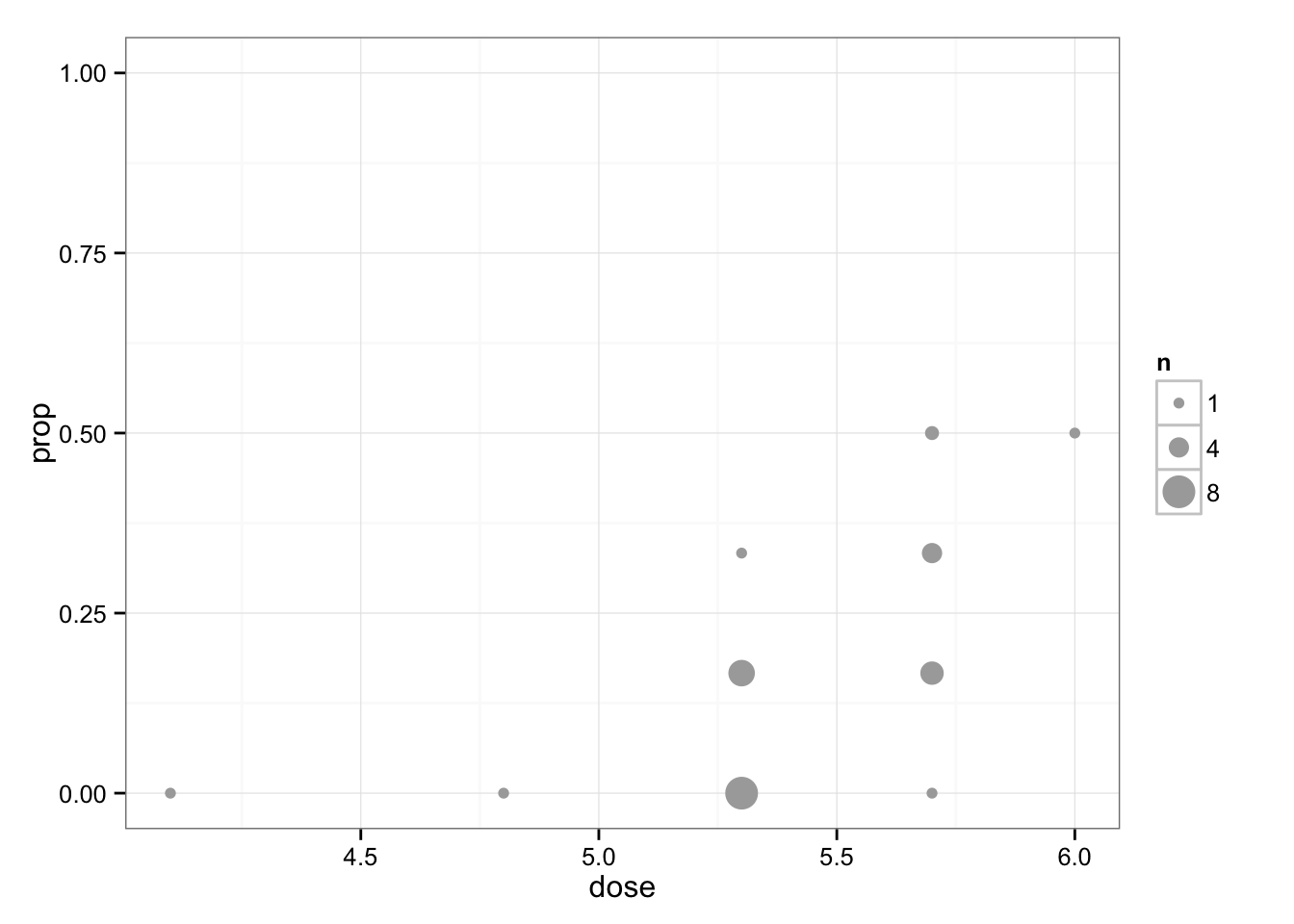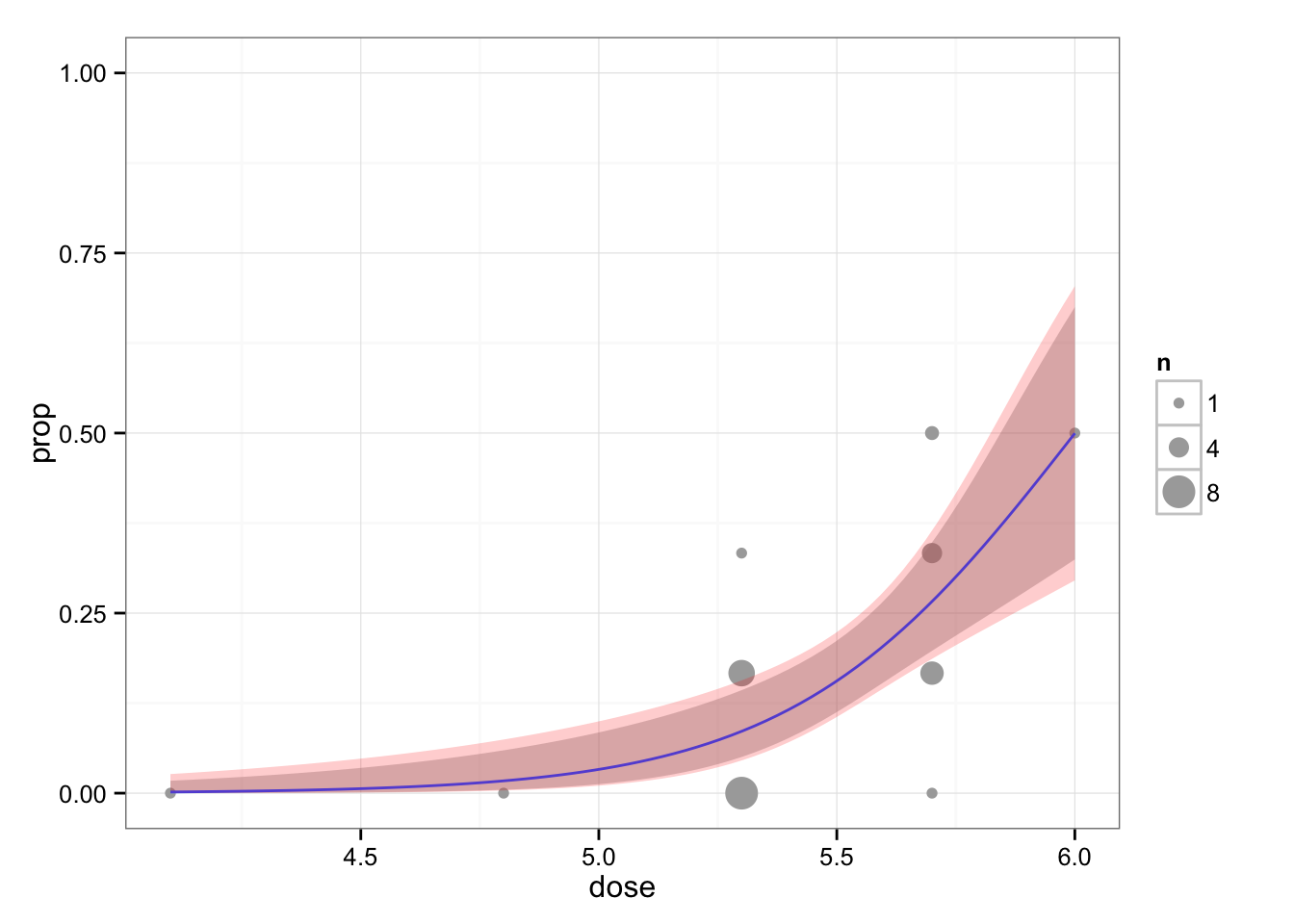## glmer offset issues

Exploring an issue with glmer and offsets, asked on r-sig-mixed-models.

tl; dr there is an initial problem with specifying offsets that give probabilities very close to 1 for the starting parameter values; this can probably be fixed in the code, but (1) it’s not too hard to adjust the offsets to fit an equivalent model; (2) it seems as though a simpler model might be adequate in this case anyway.

library("lme4")
packageVersion("lme4")
##  '1.1.8'
library("ggplot2"); theme_set(theme_bw())
library("dplyr")
slope <- 1.5

The next bit of code is what the original poster tried (I have simplified a little bit, using default Laplace approximation instead of Gauss-Hermite quadrature (nAGQ=10), to simplify/because it didn’t seem to make a difference): it fails (although it has been reported to work on one Windows machine).

try(glmer(DLT ~ offset(slope*dose) + (1 | patid),
family=binomial,data=dd))

(I am not evaluating this chunk because, even with try() and error=TRUE, it causes a segmentation fault and crashes within the RStudio/rmarkdown/render environment.) I tried setting verbose=100; this indicated that the deviance had gone to NaN very quickly, but didn’t tell us much else useful.

The proximal problem is that with the range of doses in the data (4-6) and the starting value of the intercept parameter (0), we get very high predicted probabilities (minimum of logistic(4*1.5)=0.998). I tried setting start=list(theta=1,beta=-10), but it didn’t help.

I would have thought we could go into the C++ code and make sure that the maximum predicted value for the binomial inverse-link function is bounded at 1-epsilon instead of being allowed to go to 1, but it looks like that’s done already (in glmFamily.cpp, see the logitinv and logitmueta functions) – unless we somehow missed the relevant case.

In the meantime, let’s explore a little bit more. In principle, we should be able to shift the dose variable in the offset and get equivalent models. That is, if we have a model which is $$\beta_0 + \hat \beta_1 s$$, where $$\hat \beta_1$$ is the offset value for the slope, changing it to $$\beta_0 + \hat \beta_1 (s-C)$$ is just equivalent to shifting the estimated value of $$\beta_0$$ by $$\hat \beta_1 C$$.

Shifting the dose downward 3 or by 4, these models both fit …

g1 <- glmer(DLT ~ offset(slope*I(dose-4)) + (1 | patid),
family=binomial,data=dd, nAGQ=10)
g2 <- glmer(DLT ~ offset(slope*I(dose-3)) + (1 | patid),
family=binomial,data=dd, nAGQ=10)

And give equal log-likelihoods and equivalent (different by the predicted amount of 1.5) values of the intercept $$\beta_0$$:

all.equal(fixef(g1)-1.5,fixef(g2))
##  TRUE
all.equal(logLik(g1),logLik(g2))
##  TRUE

Shifting the dose by only 2.0 units rather than 3.0 fails.

One problem with these models is that the estimated random-effects variance is zero (i.e. either data are just too small or noisy, or there is systematically less variation among groups than expected for some reason).

What happens if we try to fit the full model?

g4 <- glmer(DLT ~ dose +
## offset(1.5*I(dose-4)) +
(1 | patid),
family=binomial,data=dd, nAGQ=10)
confint(g4,which="dose")
## Computing profile confidence intervals ...
##         2.5 % 97.5 %
## dose 1.618378  5.372

We get a higher estimated value of the dose – 95% profile confidence intervals don’t even include the nominal value of 1.5 …

As usual, we should actually plot the data and see what’s going on. There are 180 total observations, with 30 patient IDs (balanced, with a single dose per patient). Since there are no observation-specific covariates, there doesn’t seem to be any compelling reason not to group the data by patient:

ddsum <- dd %>% group_by(patid) %>%
summarise(dose=dose,tot=n(),prop=mean(DLT))
(gg1 <- ggplot(ddsum,aes(x=dose,y=prop)) + ## stat_sum(aes(size=..n..))+
stat_sum(data=ddsum,aes(size=..n..),colour="darkgray")+
scale_size_continuous(range=c(2,6),breaks=c(1,4,8))+
scale_y_continuous(limits=c(0,1)))Once we’ve done that, we can easily fit a standard binomial GLM. If we want to account for among-patient variability, we can use a quasi-binomial fit. In this case, there is actually underdispersion (consistent with the variance estimate of zero above, which would be negative if it were allowed); that shows up in the following figure as a slightly larger confidence interval for the binomial (pink) than for the quasi-binomial (gray) model. (The predicted line is the same for both models.)

gg1 + geom_smooth(method="glm",aes(weight=tot),
family="quasibinomial")+
geom_smooth(method="glm",aes(weight=tot),
alpha=0.2,
family="binomial",fill="red",colour=NA)This seems to capture the data reasonably well.